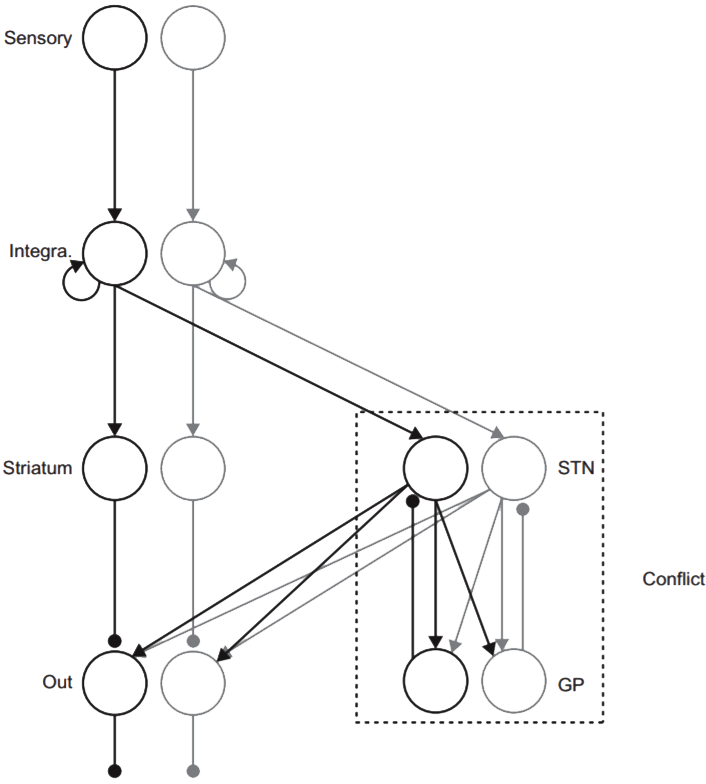## Simulation Codes: Optimal decision-making theories

Bogacz R
Description

This compressed file includes 6 files containg Matlab scripts. You can get help on each file by typing in Matlab "help filename". As will be explained in detail below, the files allow comparing the decision time (DT) of different models of choice for fixed required error rate (ER). Hence they automatically find the decision threshold giving the required ER of the model, and then calculate DT for this threshold.

To be able to use the above mentioned functions, the model needs to be defined as a single Matlab function of the following format:

`[er,rt] = model (z, param);`

Hence the function needs to take two parameters: z - the decision threshold, and param - vector of additional parameters, and the function should return the mean ER and DT. Three examples of functions in this format are provided by files mutinh.m, feedinh.m and MSPRT.m, that implement the mutual inhibition model (Usher and McClelland, 2001), the feedforward inhibition model (Shadlen and Newsome, 2001) and the MSPRT model (Bogacz and Gurney, 2007). After typing "help mutinh" the following message appears:

function [er,rt] = mutinh (z, param) Simulation of mutual inhibition model (100 simulated decisions) Inputs: z - decision threshold param - vector of other parameters: param(1) = I1 - mean input to the first integrator param(2) = I2 - mean input to the second integrator param(3) = c - r.m.s. mangnitude of noise in the input param(4) = k - decay rate of integrators param(5) = w - weight of mutual inhibition Outputs: er - mean error rate rt - mean decision time

The reason why all the additional parameters are stored in the vector is to allow finding threshold for different models having different numbers and types of parameters. The main function which finds the DT for required ER is RTforER10.m, which has the following format:

`function [rt, sert, er, mz] = RTforER10 (model, param {, reqER})`

It requires two parameters: the name of the function describing the model, and the model's parameters. By default, it finds the DT for ER of 10% (as suggested by its name), if different ER is required, it can be specified by the third parameter. Hence for example, to find DT of mutual inhibition model with I1=4.5, I2=3, c=0.33, k=w=10 for threshold giving ER=10%, type in Matlab:

`param = [4.5, 3, 0.33, 10, 10]; RTforER10 ('mutinh', param)`

If you require error bars showing standard error, you can use function `repeatedRT.m` which simply executes RTforER10 multiple times. To find out about details, type in Matlab `help repeatedRT`.

Have fun!

Assistance with this dataset

We welcome researchers wishing to reuse our data to contact the creators of datasets. If you are unfamiliar with analysing the type of data we are sharing, have questions about the acquisition methodology, need additional help understanding a file format, or are interested in collaborating with us, please get in touch via email. Our current members have email addresses on our main site. The corresponding author of an associated publication, or the first or last creator of the dataset are likely to be able to assist, but in case of uncertainty on who to contact, email Ben Micklem, Research Support Manager at the MRC BNDU.Year Published
2018
Publisher
University of Oxford